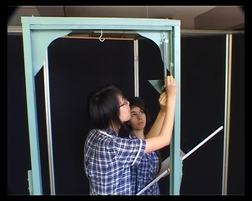PLAY PREVIEW

### Segments in this Video

#### Mathematical Relationships(01:49)

FREE PREVIEW

The physical world can be described by mathematical relationships. Understanding the relationships allows scientists to predict behavior. Edwin Hubble found the relationship between the velocity other galaxies are moving away from our galaxy and their distance.

#### Measurement and the Search for Relationships(01:32)

Students conduct an experiment to find the period of oscillation, or T, for a steel spring. The period of oscillation is the dependent variable. The mass on the spring is the independent variable.

#### The Effect on T of Mass (First Independent Variable)(04:47)

The students are keeping the spring's tightness constant to see the effects mass has on the period of oscillation, or T. They test for T using different masses.

#### Spring Stiffness (k)(01:54)

The students determine the relationship between the period of oscillation and the stiffness of the spring. They measure stiffness by testing the spring with different masses and plotting force against extension.

#### The Effects on T of Spring Stiffness (second independent variable)(02:34)

The students test how spring stiffness effects the period of oscillation. They put the same mass on springs with different stiffness. The mathematical form of the graph is not obvious and must be analyzed.

#### Combining Two Relationships(02:24)

The students have determined the period of oscillation's relationship with mass and spring stiffness. The students combine the two independent variables into one equation. They use their data to find the value for the unknown variable.

Scientific experiment must be recorded in reports so there is a record of what was done to produce the data. The students write the aim, apparatus, method, results, analysis, discussion, and conclusion for the report.

#### Credits: Measurement and the Search for Relationships(00:33)

Credits: Measurement and the Search for Relationships

For additional digital leasing and purchase options contact a media consultant at 800-257-5126
(press option 3) or sales@films.com.

# Measurement and the Search for Relationships

 DVD (Chaptered) Price: \$129.95 DVD + 3-Year Streaming Price: \$194.93 3-Year Streaming Price: \$129.95

Share

### Description

Presenting a case study, this program teaches how an experiment can be designed to test the effects of two independent variables. It also looks at how mathematical formulas can be derived from experimental measurements.

Length: 20 minutes

Item#: BVL150915

ISBN: 978-1-64347-745-9Closed Captioned